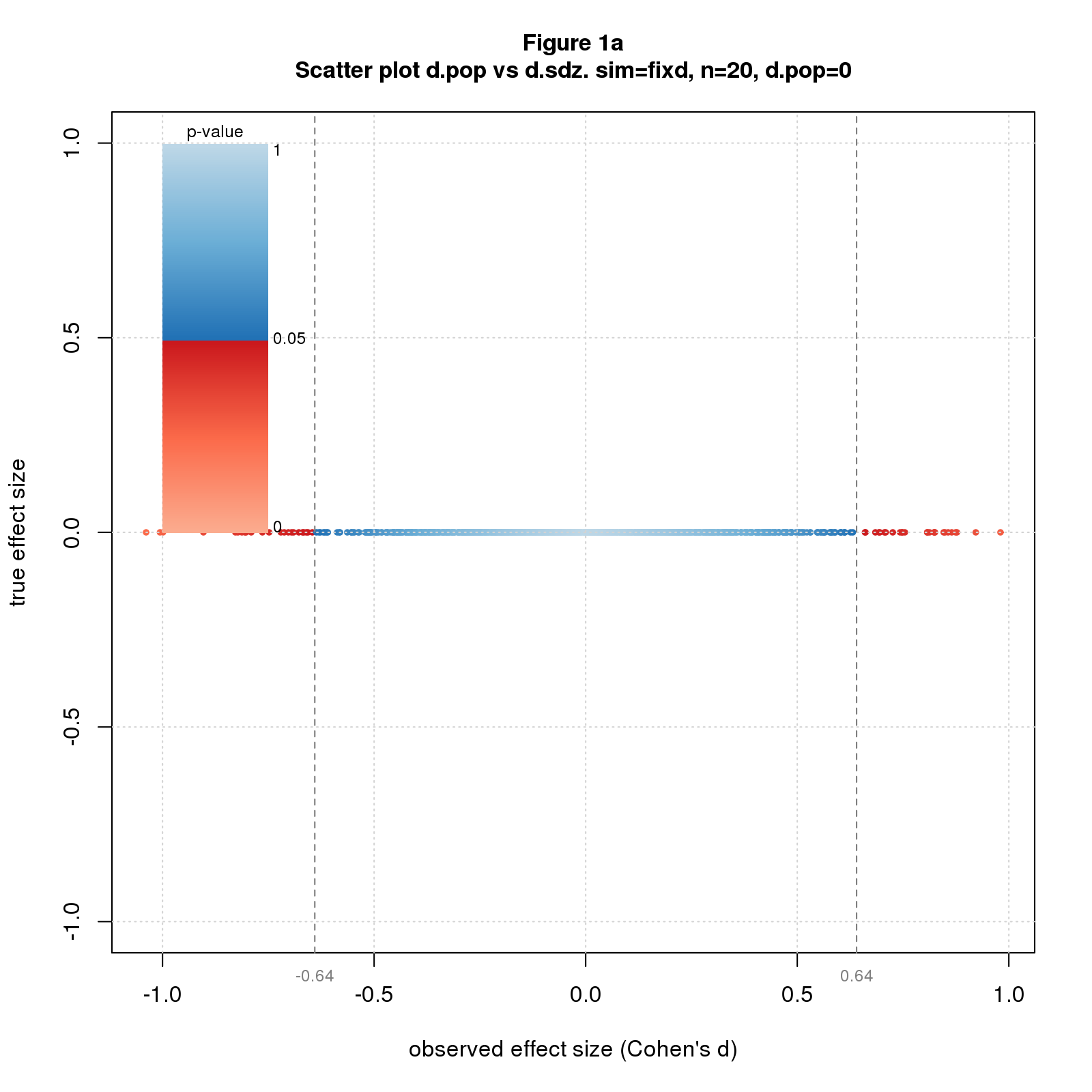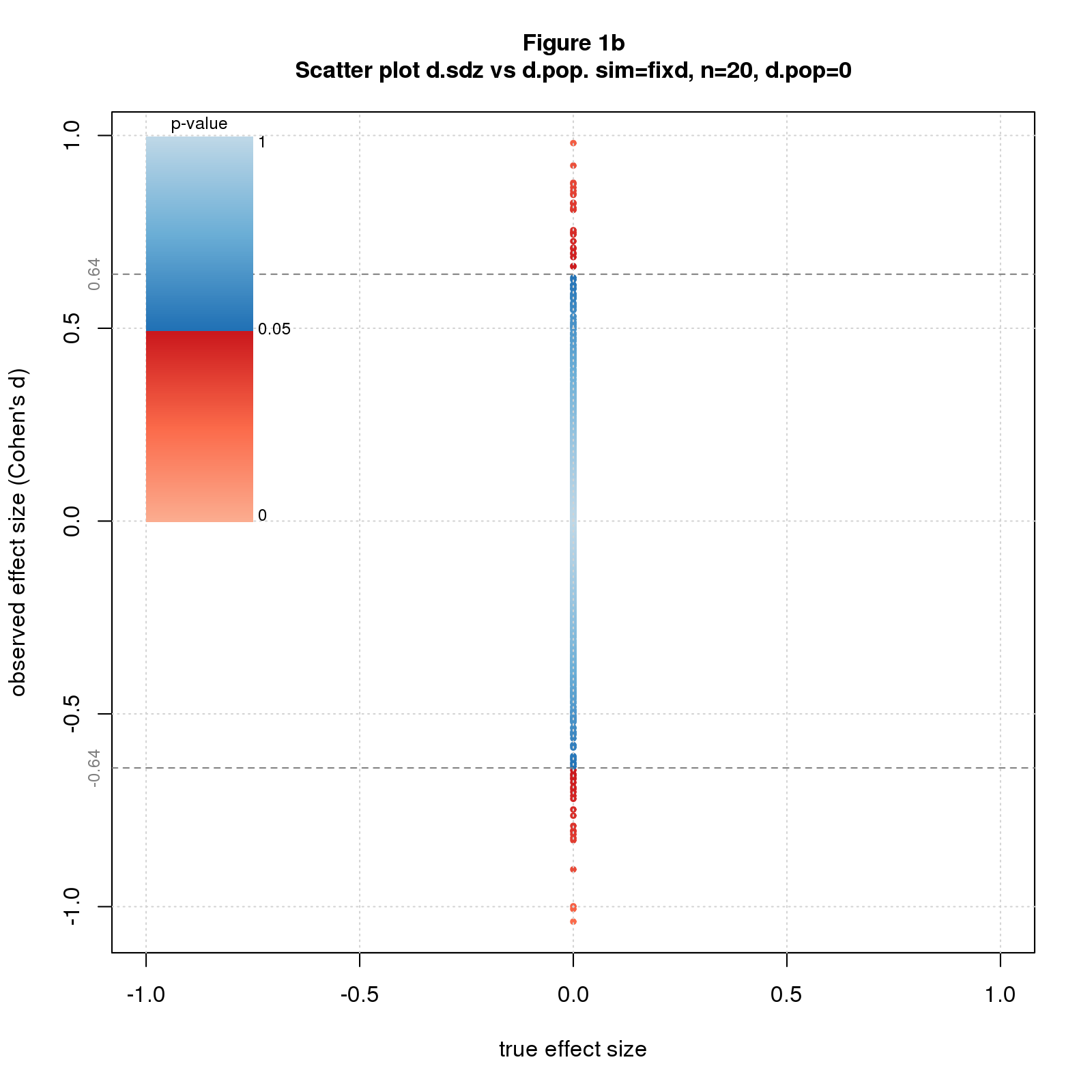## Introduction

This document contains supplementary material for the README document associated with this repo. It’s not meant to be read as a standalone document. It’s more of a “usage test” demonstrating that all major functions work in the limited setting of this document. It proved it’s worth in this regard, driving out several bugs and leading to software improvements.

The document exercises all capabilities of the program: simulation and other data generation, plotting of figures, and saving of summary results. It has figures showing each plot function applied to each kind of data that can be sensibly displayed using that function. Some figures are included for completeness even though they may not be terribly informative. It also illustrates custom plots from the blog posts.

The figures as displayed in the document are tiny - drawn at 25% scale - to reduce screen real estate. The underlying image data is full scale, however, and readers can expand the figures if desired.

The simulations driving the figures too small to yield stable results - $$10^3$$ iterations vs. $$10^4$$ or $$10^5$$ in the blog posts. This explains why some of the figures are so jumpy.

## Overview

Nomenclature

in code in text meaning
n $$n$$ sample size
d.pop $$d_{pop}$$ population effect size
d.sdz $$d_{sdz}$$ standardized observed effect size, aka Cohen’s d
d.het $$d_{het}$$ mean of heterogeneous population distribution
d0 $$d0$$ “center” of non-central t-distribution, more precisely, the non-centrality parameter (ncp) expressed in d units; equivalently, the presumed true effect size for which this is the sampling distribution
d $$d$$ variously means either $$d_{pop}$$, $$d_{sdz}$$, or $$d_{het}$$; hopefully clear from context
sd.het $$sd_{het}$$ standard deviation of heterogeneous population distribution
meand $$meand$$ mean significant observed effect size
pval $$pval$$ p-value
power $$power$$ power
ci $$ci$$ confidence interval
simu $$simu$$ statistic computed from simulated data
theo $$theo$$ statistic computed analytically

The program implements two data generation models.

1. fixed effect (fix) which imagines we’re repeating a study an infinite number of times, drawing a different sample each time from a population with a fixed true effect size
2. heterogeneous effect (het) which assumes that each time we do the study, we’re sampling from a different population with a different true effect size

The program explores the models with three types of simulations.

1. fixd. Fixes $$d_{pop}$$ to a few values of interest, sets $$n$$ to a range of values, and simulates many studies for each $$d_{pop}$$ and $$n$$.
2. rand. Randomly selects a large number of $$d_{pop}$$s, sets $$n$$ to a range of values, and simulates one study for each $$d_{pop}$$ and $$n$$.
3. hetd. Sets $$d_{het}$$ and $$sd_{het}$$ to a few values of interest, and selects a large number of $$d_{pop}$$s from normal distributions with mean $$d_{het}$$ and standard deviation $$sd_{het}$$. Then sets $$n$$ to a range of values, and simulates one study for each $$d_{pop}$$ and $$n$$.

The program calculates four statistics across the data generation models and simulation types.

1. meand (mean significant effect size)
2. p-values
3. power
4. confidence intervals

## plotdvsd - Plot one effect size vs another

The first group of figures are scatter plots colored by p-value of $$d_{pop}$$ vs $$d_{sdz}$$ and vice versa for all three simulation types. The program plots results for two parameter sets and has full scale and zoomed-in figures. The zoomed-in figures illustrate the sharp boundary between significant and non-significant results.

Technical note: The sharpness of the boundary is due to the use of Cohen’s d in conjunction with the t-test. This pairing is mathematically natural because both are standardized, meaning both are relative to the sample standard deviation. In fact, Cohen’s d and the t-statistic are essentially the same statistic, related by the identities $$d=t\sqrt{2/n}$$ and $$t=d\sqrt{n/2}$$ (for my simulation scenario).

There are blocks of eight figures for each type of simulation. The fixd figures aren’t very informative since there’s no $$d_{pop}$$ spread; I include them for completeness only. You’ll notice the often-poor placement of the p-value legend; this is a limitation of the program.

fixd simulation